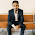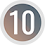# Percentage | What is percentage?## Percentage | What is percentage?

Percentage is the rate per hundred and percent means per hundred i.e. out of hundred. For example 15 percent means 15 out of 100. A percentage is a fraction having the denominator 100. The symbol to denote percent is % of any fraction. Say, 4/25 is equivalent to 16/100 which denotes 16%.

#### To convert fraction or decimal into percent:

To convert a fraction or decimal into percent we have to multiply by 100%.
For example: 3/10 = 3/10 × 100% = 30%
Similarly,
0.36 = 0.36 × 100% = 36%

#### To convert a percent into fraction:

To convert a percent into fraction we have to divide by 100.
For example: 46% = 46/100 = 23/50
Similarly,
7.5% = 7.5/100 = 75/1000 = 3/40

#### To find the percent of quantities:

To find the percent of quantities we convert the percent into fraction and multiply with total quantity.
For example: Take 32% of Rs. 75
= 32/100 × Rs. 75
= Rs. 24

### Workout Examples

Example 1: Among 225 candidates, 20% failed. Find the number of candidates who passed.

Solution: Here,
Total candidates = 225

Failed = 20% of 225
= 20/100 × 225
= 45

Passed = Total – failed
= 225 – 45
= 180

Example 2: A boy gets 25 marks out of 75. What percent of marks does he get?

Solution: Here,
A boy gets 25 out of 75

He got  = 25/75 × 100%
= 100/3%
= 33.33%

Example 3: There are 6000 mangoes in a fruit shop, among them 1500 are sold. What percent of mangoes are unsold?

Solution: Here,
Total mangoes = 6000
Sold mangoes = 1500
Unsold mangoes  = 6000 – 1500
= 4500

Unsold percent  = 4500/6000 × 100%
= 25%

Example 4: 25% of total students in a school are boys. If 150 students were girls, find the number of boys.

Solution: Here,
Percent of boys = 25%
Percent of girls = 100 – 25% = 75%
No. of girls = 150
Let, total students = x

75% of x = 150
or,        75/100 × x = 150
or,        x = 150 × 100/75
or.        x = 200

Total students = 200

No. of boys = 200 – 150
= 50

Example 5: The price of petrol is increased from Rs. 105 per litre to Rs. 125 per litre, what percent is increased?

Solution: Here,
Increased price = Rs. 125 – 105
= Rs. 20

Increased percent = 20/105 × 100%
= 100/7%
= 19.05%

You can comment your questions or problems regarding the percentage here.

1.in an election between two candidates, 20% of the registered voters did not cast their vote and 40 votes were declared invalid. the winner received 42% of the registered votes and was declared the winner by 112 votes. what was the number of registered voters.

1.Solution:
Let, no of registered voters = x
Did not cast = 20% of x = 20x/100